Posted on

## Car detection in videosPeter Horvath

We detect cars from videos recorded by dash cameras situated in cars. This type of camera is dynamic so we decided to train and use Haar Cascade Classifier. The classifier itself returns a lot of false positive results. So we improved classifier by removing false positive results using road detection.

Functions used:Â cvtColor, split, Rect, inRange, equalizeHist, detectMultiScale, rectangle, bitwise_and

## Process

1st part â€“ training haar cascade classifier

Collect aÂ set of positive samples and negative samples. Make aÂ list file of both (positives.dat and negatives.dat). Then use opencv_createsamples function with parameters to make aÂ single .vec file with all positive samples.

```opencv_createsamples -info positives.dat -vec samples.vec -num 500 -w 20 -h 20
```

Now train aÂ cascade classifier using HAAR features

```opencv_traincascade -data classifier -featureType HAAR -vec samples.vec -bg negatives.dat -numPos 500 -numNeg 850 -numStages 15 -precalcValBufSize 1000 -precalcIdxBufSize 1000 -minHitRate 0.999 -maxFalseAlarmRate 0.5 -mode ALL -w 20 -h 20
```

Output of this procedure is trained classifier â€“ xml file.

2nd part â€“ using classifier in C++ code to detect cars, improved by road detection

Open video file using VideoCapture. For every video frame do:

1. Convert actualÂ video frame to HSV color model
```cvtColor(frame, frame_hsv, CV_BGR2HSV);
```
2. Make sum of H S V in captured road sample. Calculate average Hue Saturation and Value of captured road sample.
```int averageHue = sumHue / (rectangle_hsv_channels.rows*rectangle_hsv_channels.cols);
int averageSat = sumSat / (rectangle_hsv_channels.rows*rectangle_hsv_channels.cols);
int averageVal = sumVal / (rectangle_hsv_channels.rows*rectangle_hsv_channels.cols);
```
3. Use inRange function to make a binary result â€“ road is white colored, other is black colored
```inRange(frame_hsv, cv::Scalar(averageHue - 180, averageSat - 15, averageVal - 20), cv::Scalar(averageHue + 180, averageSat + 15, averageVal + 20), final);
```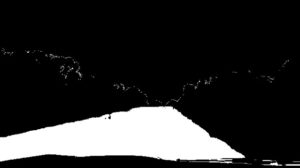4. Convert actual video frame to grayscale
```cvtColor(frame, image_gray, CV_BGR2GRAY);
```
5. Create an instance of CascadeClassifier
```String car_cascade_file = "classifier.xml";
```
6. Detect cars in grayscale video frame using classifier
```car_classifier.detectMultiScale(image_gray, cars, 1.1, 2, 0 | CV_HAAR_SCALE_IMAGE, Size(20, 20));
```

Result have a lot of false positives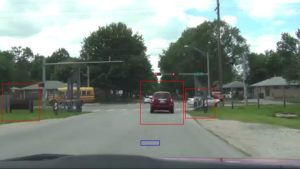7. Make a black image with white squares at locations returned by cascade classifier. Make logical and between it and image with detected road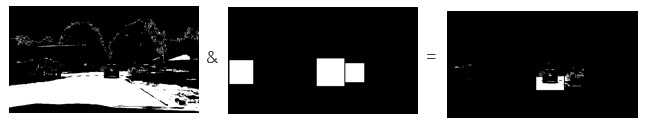8. Accept only squares which have at least 20% of pixels white.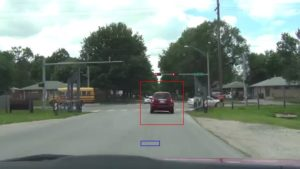Limitations:

• Cascade classifier trained only with 560 positive and 860 negative samples â€“ detect cars only from near distance
• Road detection fails when some object (car, road line) comes to blue rectangle (supposed to be road sample)
• Dirt have a similar saturation as road â€“ detected as road
Posted on

## Card detectionMichael Garaj

The goal of this project is to detect card in captured image. Motivation was to make automatized recognizer of cards for poker tournaments. Application is implemented to find orthogonal edges in an image and try to find card by ratio of its edges.

Process of finding and recognizing a card in image follows these steps:

1. Load an image from local repository.
2. Apply blur and bilateral filter.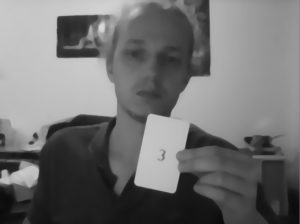3. Compute binary threshold.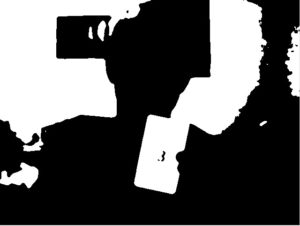4. Extract edges from binary image by Canny algorithm.
5. Apply Hough lines to get lines find in edge image.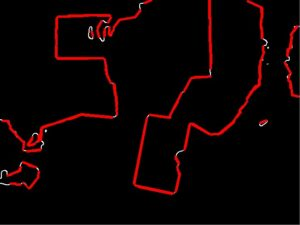6. Search for orthogonal lines and store them in structure for future optimalization.
7. Optimise number of detected lines in same area by choosing only the biggest ones.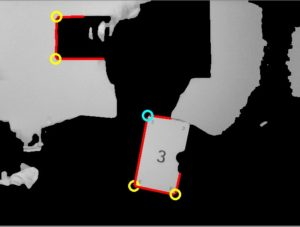8. Find card which consist of 3 touching lines.
9. Compute ratio of the lines and identify cards in the image.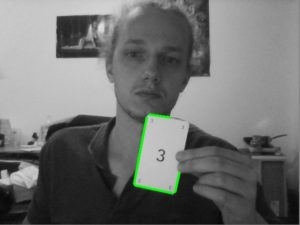Following code sample shows steps of optimalization of detected corners:

```vector<MyCorner> optimalize(vector<MyCorner> corners, Mat image) {
vector<MyCorner> optCorners;

for (int i = 0; i < corners.size(); i++) {
corners[i].crossing = crossLines(corners[i]);
corners[i].single = 1;
}

int distance = 25;
for (int i = 0; i < corners.size() - 1; i++) {
MyCorner corner = corners[i];
float lengthI = 0, lengthJ = 0;

if (corner.single){
for (int j = i + 1; j < corners.size(); j++) {

if (abs(corner.crossing.x - corners[j].crossing.x) < distance && abs(corner.crossing.y - corners[j].crossing.y) < distance &&
(corner.single || corners[j].single)) {

lengthI = getLength(corner.u) + getLength(corner.v);
lengthJ = getLength(corners[j].u) + getLength(corners[j].v);

if (lengthI < lengthJ) {
corner = corners[j];
}
corner.single = 0;
corners[i].single = 0;
corners[j].single = 0;
}
}
optCorners.push_back(corner);
}
}

return optCorners;
}
```
Posted on

## Bag of Words algorithm

Tomas Drutarovsky

We implement well-known Bag of Words algorithm (BoW) in order to perform image classification of tiger cat images. In the work, we use a subset of publicly available ImageNet dataset and divide data on two sets â€“ tiger cats and non-cat objects, which consist of images of 10 random chosen object types.

The main processing algorithm is performed by these steps:

1. Choose a suitable subset of images from a large dataset
• We use around 100 000 unique images
2. Detect keypoints
• We detect keypoints using SIFT or Dense keypoint extractor
```DenseFeatureDetector dense(20.0f, 3, 2, 10, 4);
BOWKMeansTrainer bowTrainer(dictionarySize, tc, retries, flags);

for (int i = 0; i < list.count(); i++){

dense.detect(img, keypoints);
}
```Keypoints detected using SIFT detect function – more than 500 keypoints.
3. Describe keypoints using SIFT
• SIFT descriptor produces description for each keypoint separately
```sift.compute(img, keypoints, descriptor);
```
4. Cluster descriptors using k-means
• Around 10 million of keypoints are chosen to cluster
• Clustering results in 1000 clusters represented by centroids (visual words)
```Mat vocabulary = bowTrainer.cluster();
```
5. Calculate BoW descriptors
• Each keypoint from an input image is then evaluated for response from 1000 visual words or represents
• Histogram of reponse is normalized for each image
```Ptr<DescriptorMatcher> matcher(new FlannBasedMatcher);
Ptr<FeatureDetector> detector(new SiftFeatureDetector());
BOWImgDescriptorExtractor bowExtractor(detector, matcher);
bowExtractor.compute(img, keypoints, descriptor);
```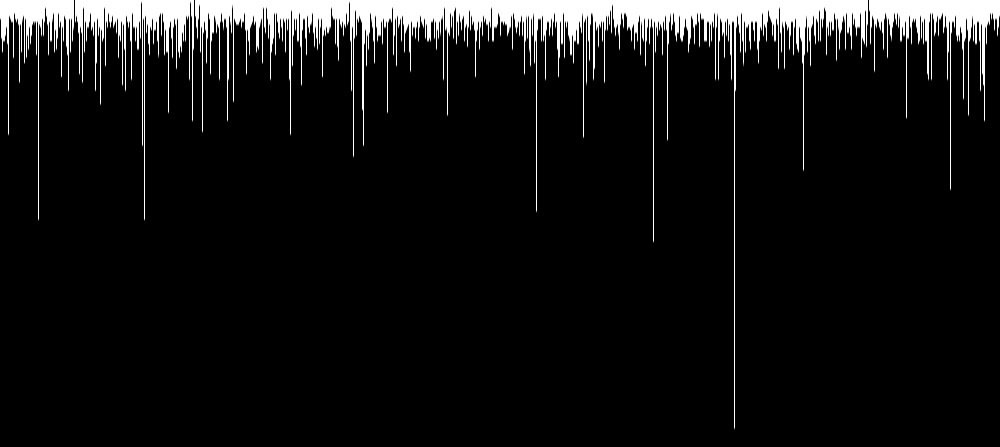BoW descriptor of 200 ats visualized over 1000 clustered visual words vocabulary
6. Train SVM using BoW descriptors
• Calculated histograms or BoW descriptors are trained using linear SVM
• Suitable rate between positive and negative subset needs to be chosen
7. Test images using SVM
• Response of test images is used to evaluate algorithm
• Our model shows accuracy of (62% of positive set and 58% of negative set)
• Better results are achievable using larger datasets, but both time and computational power are necessary
Posted on

## Face recognition using depth data from Kinect sensor

We will segment face from color camera with use of depth data and run recognition on it using OpenCV functions: EigenFaces, FisherFaces and LBPH.

Complete process is as follows:

1. First we need to obtain RGB and depth stream from Kinect sensor and copy it to byte array in order to be usable for OpenCV
```IColorFrame* colorFrame = nullptr;
IDepthFrame* depthFrame = nullptr;
ushort* _depthData = new ushort[depthWidth * depthHeight];
byte* _colorData = new byte[colorWidth * colorHeight * BYTES_PER_PIXEL];

colorFrame->CopyConvertedFrameDataToArray(colorWidth * colorHeight * BYTES_PER_PIXEL, _colorData, ColorImageFormat_Bgra);
depthFrame->CopyFrameDataToArray(depthWidth * depthHeight, _depthData);

```
2. Because color and depth camera have different resolutions we need to map coordinates from color image to depth image. (We will use Kinectâ€™s Coordinate Mapper)
```m_pCoordinateMapper->MapDepthFrameToColorSpace(depthWidth * depthHeight,(UINT16*) _depthData, depthWidth * depthHeight, _colorPoints);
```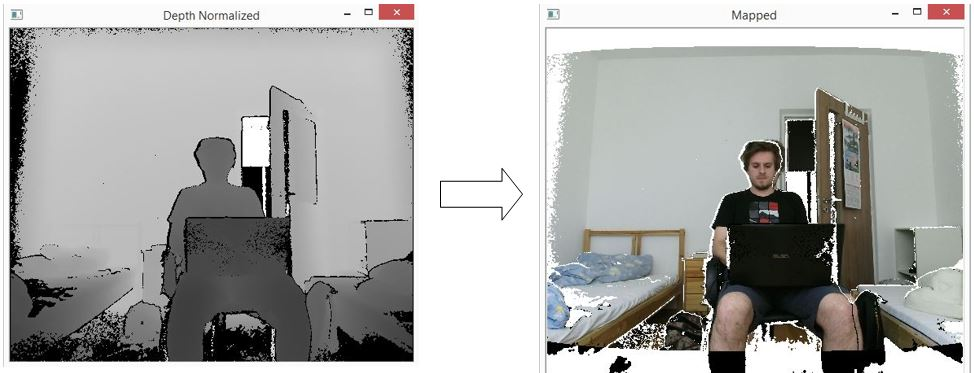3. Because we are going to segment face from depth data we need to process them as is shown in the next steps:
1. Unmodified depth data shown in 2D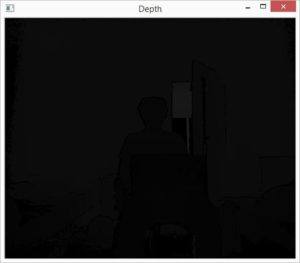2. Normalization of values to 0-255 range
â€“Â Better representation

```cv::Mat img0 = cv::Mat::zeros(depthHeight, depthWidth, CV_8UC1);
double scale = 255.0 / (maxDist - minDist);
depthMap.convertTo(img0, CV_8UC1, scale);
```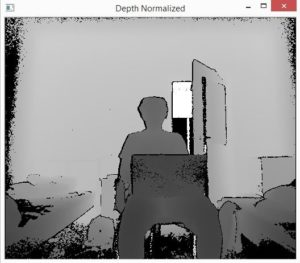3. Removal of the nearest points and bad artifacts
â€“Â the points for which Kinect canâ€™t determine depth value are by default set to 0 â€“ we will set them to 255

```if (val < MinDepth)
{
image.data[image.step * i + image.step * j + 0] = 255;
}

```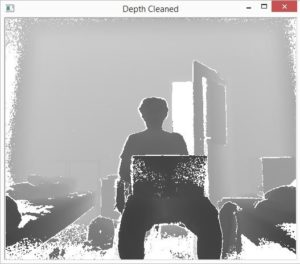4. Next we want to segment person, we apply depth threshold to filter only nearest points and the ones within certain distance from them and apply median blur to image to remove unwanted artifacts such as isolated points and make edges of segmented person less sharp.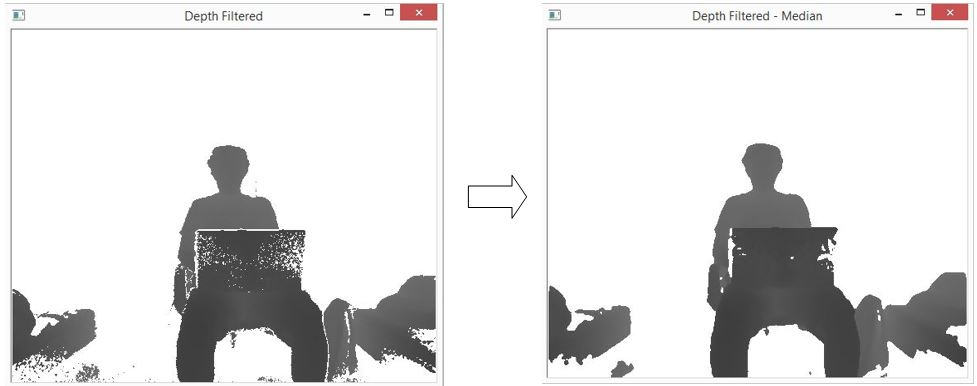```if (val > (__dpMax+DepthThreshold))
{
image.data[image.step * i + image.step * j + 0] = 255;
}
```
4. Now when we have processed depth data we need to segment face. We find the highest non-white point in depth map and mark it as the top of head. Next we make square segmentation upon depth mask with dynamic size (distance from user to sensor is taken into account) from top of the head and in this segmented part we find the leftmost and rightmost point and made second segmentation. The 2 new points and point representing top of the head will now be the border points of the new segmented region. (Sometimes because of dynamic size of square we have also parts of shoulders in our first segmentation, in order to mitigate this negative effect we are looking for leftmost and rightmost point only in the upper half of the image)
```if (val == 255 || i > (highPointX + headLength) || (j < (highPointY - headLength / 2) && setFlag) || (j > (highPointY + headLength / 2) && setFlag))
{
//We get here if point is not in face segmentation region
...
}
else if (!setFlag)
{
//We get here if we find the first non-white (highest) point in image and set segmentation region
highPointX = i;
highPointY = j;
headLength = 185 - 1.2*(val); //size of segmentation region
setFlag = true;
...
}
else
{
//We get here if point is in face segmentation region and we want to find the leftmost and the rightmost point

if (j < __leftMost && i < (__faceX + headLength/2)) __leftMost = j;
if (j > __rightMost && i < (__faceX + headLength/2)) __rightMost = j;
}

```
5. When face is segmented we can use one of OpenCV functions for face recognition and show result to the user.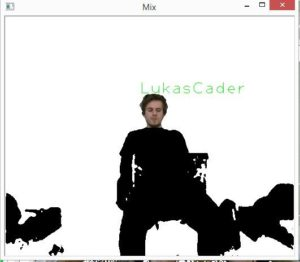Posted on

## Pedestrian detection

This project focuses on preprocessing of training images for pedestrian detection. The goal is to train a model of a pedestrian detection. Histogram of oriented gradients HOG has been used as descriptor of image features. Support vector machine SVM has been used to train the model.

Example:

There are several ways to cut train example from source:

1. using simple bounding rectangle
2. adding â€œpaddingâ€ around simple bounding rectangle
3. preserve given aspect ratio
4. using only upper half of pedestrian body

At first, the simple bounding rectangle around pedestrian has been determined. Annotation of training dataset can be used if it is available. In this case a segmentation annotation in form of image mask has been used. Bounding box has been created from image mask using contours (if multiple contours for pedestrian has been found, they were merged to one).);

```findContours(mask, contours, CV_RETR_TREE, CV_CHAIN_APPROX_SIMPLE);
boundRect[k] = boundingRect(Mat(contours[k]));
```

HOG descriptors has been computed using OpenCV function hog.compute(), where descriptor parameters has been set like this:

```Size win_size = Size(64, 128);
HOGDescriptor hog = HOGDescriptor(win_size, Size(16, 16), Size(8, 8), Size(8, 8), 9);
```

Window width = 64 px, window height = 128 px, block size = 16×16 px, block stride = 8×8 px, cell size = 8×8 px and number of orientation bins = 9.

Each input image has been re-scaled to fit the window size.

1.) Image, which had been cut using simple bounding rectangle, has been resizedÂ to fit aspect ratio of descriptor window.

2.) In the next approach the simple bounding rectangle has been enlarged to enrich descriptor vector with background information. Padding of fixed size has been added to each side of image. When the new rectangle exceeded the borders of source image, the source image has been enlarged by replication of marginal rows and columns.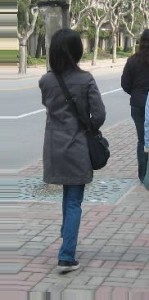```if (params.add_padding)
// Apply padding around patches, handle borders of image by replication
{
if (l < 0)
{
int addition_size = -l;
copyMakeBorder(timg, timg, 0, 0, addition_size, 0, BORDER_REPLICATE);
l = 0;
}
if (t < 0)
{
int addition_size = -t;
copyMakeBorder(timg, timg, addition_size, 0, 0, 0, BORDER_REPLICATE);
t = 0;
}
if (r > = timg.size().width)
{
int addition_size = r - timg.size().width + 1;
copyMakeBorder(timg, timg, 0, 0, 0, addition_size, BORDER_REPLICATE);
}
if (b > = timg.size().height)
{
int addition_size = b - timg.size().height + 1;
copyMakeBorder(timg, timg, 0, addition_size, 0, 0, BORDER_REPLICATE);
}
allBoundBoxesPadding[i] = Rect(Point(l, t), Point(r, b));
}
```

3. In the next approach the aspect ratio of descriptor window has been preserved while creating the cutting bounding rectangle (so pedestrian were not deformed). In this case the only necessary padding has been added.

4. In the last approach only the half of pedestrian body has been used.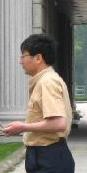```int hb = t + ((b - t) / 2);
allBoundBoxes[i] = Rect(Point(l, t), Point(r, hb));
```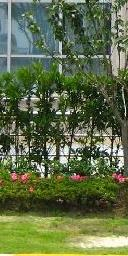```NG rng(12345);
static const int MAX_TRIES = 10;
int examples = 0;
int tries = 0;
int rightBoundary = img.size().width - params.neg_example_width / 2;
int leftBoundary = params.neg_example_width / 2;
int topBoundary = params.neg_example_height / 2;
int bottomBoundary = img.size().height - params.neg_example_height / 2;
```
```while (examples < params.negatives_per_image && tries < MAX_TRIES)
{
int x = rng.uniform(leftBoundary, rightBoundary);
int y = rng.uniform(topBoundary, bottomBoundary);
bool inBoundingBoxes = false;
for (std::vector::iterator it = allBoundBoxes.begin();
it != allBoundBoxes.end();
it++)
{
if (it->contains(Point(x, y)))
{
inBoundingBoxes = true;
break;
}
}
if (inBoundingBoxes == false) {
Rect rct = Rect(Point((x - params.neg_example_width / 2), (y - params.neg_example_height / 2)), Point((x + params.neg_example_width / 2), (y + params.neg_example_height / 2)));
boost::filesystem::path file_neg = (params.negatives_target_dir_path / img_path.stem()).string() + "_" + std::to_string(examples) + img_path.extension().string();
imwrite(file_neg.string(), img(rct));
examples++;
}
tries++;
}
```

SVM model has been learned using Matlab function fitcsvm(). The single descriptor vector has been computed:

```ay = SVMmodel.Alpha .* SVMmodel.SupportVectorLabels;
sv = transpose(SVMmodel.SupportVectors);
single = sv*ay;
% Append bias
single = vertcat(single, SVMmodel.Bias);
% Save vector to file
dlmwrite(model_file, single,'delimiter','\n');
```

Single descriptor vector has been loaded and set
( hog.setSVMDetector(descriptor_vector) ) in detection algorithm which used the OpenCV function hog.detectMultiScale() to detect occurrences on multiple scale within whole image.

HOG visualization
As a part of project, the HOG descriptor visualization has been implemented. See algorithm bellow. Orientations and magnitudes of gradients are visualized by lines at each position (cell). In first part of algorithm all values from normalization over neighbor blocks at given position has been merged together. Descriptor vector of size 9×4 for one position has yielded vector of size 9.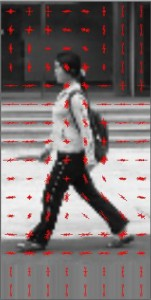```void visualize_HOG(std::string file_path, cv::Size win_size = cv::Size(64, 128), int
visualization_scale = 4)
{
using namespace cv;
// resize image (size must be multiple of block size)
resize(img, img, win_size);
HOGDescriptor hog(win_size, Size(16, 16), Size(8, 8), Size(8, 8), 9);
vector descriptors;
hog.compute(img, descriptors, Size(0, 0), Size(0, 0));
size_t cell_cols = hog.winSize.width / hog.cellSize.width;
size_t cell_rows = hog.winSize.height / hog.cellSize.height;
size_t bins = hog.nbins;
// block has size: 2*2 cell
size_t block_rows = cell_rows - 1;
size_t block_cols = cell_cols - 1;
size_t block_cell_cols = hog.blockSize.width / hog.cellSize.width;
size_t block_cell_rows = hog.blockSize.height / hog.cellSize.height;
size_t binspercellcol = block_cell_rows * bins;
size_t binsperblock = block_cell_cols * binspercellcol;
size_t binsperblockcol = block_rows * binsperblock;
struct DescriptorSum
{
vector bin_values;
int components = 0;
DescriptorSum(int bins)
{
bin_values = vector(bins, 0.0f);
}
};
vector average_descriptors = vector(cell_cols, vector(cell_rows, DescriptorSum(bins)));
// iterate over block columns
for (size_t col = 0; col < block_cols; col++)
{
// iterate over block rows
for (size_t row = 0; row < block_rows; row++)
{
// iterate over cell columns of block
for (size_t cell_col = 0; cell_col < block_cell_cols; cell_col++)
{
// iterate over cell rows of block
for (size_t cell_row = 0; cell_row < block_cell_rows; cell_row++)
{
// iterate over bins of cell
for (size_t bin = 0; bin < bins; bin++)
{
average_descriptors[col + cell_col][row + cell_row].bin_values[bin] += descriptors[(col*binsperblockcol) + (row*binsperblock) + (cell_col*binspercellcol) + (cell_row*bins) + (bin)];
}
average_descriptors[col + cell_col][row + cell_row].components++;
}
}
}
}
resize(img, img, Size(hog.winSize.width * visualization_scale, hog.winSize.height * visualization_scale));
cvtColor(img, img, CV_GRAY2RGB);
Scalar drawing_color(0, 0, 255);
float line_scale = 2.f;
int cell_half_width = hog.cellSize.width / 2;
int cell_half_height = hog.cellSize.height / 2;
double rad_per_bin = M_PI / bins;
int max_line_length = hog.cellSize.width;
// iterate over columns
for (size_t col = 0; col < cell_cols; col++)
{
// iterate over cells in column
for (size_t row = 0; row < cell_rows; row++)
{
// iterate over orientation bins
for (size_t bin = 0; bin < bins; bin++)
{
float actual_bin_strength = average_descriptors[col][row].bin_values[bin] / average_descriptors[col][row].components;
// draw lines
if (actual_bin_strength == 0)
continue;
int length = static_cast(actual_bin_strength * max_line_length * visualization_scale * line_scale);
double angle = bin * rad_per_bin + rad_per_halfbin + (M_PI / 2.f);
double yrange = sin(angle) * length;
double xrange = cos(angle) * length;
Point cell_center;
cell_center.x = (col * hog.cellSize.width + cell_half_width) * visualization_scale;
cell_center.y = (row * hog.cellSize.height + cell_half_height) * visualization_scale;
Point start;
start.x = cell_center.x + static_cast(xrange / 2);
start.y = cell_center.y + static_cast(yrange / 2);
Point end;
end.x = cell_center.x - static_cast(xrange / 2);
end.y = cell_center.y - static_cast(yrange / 2);
line(img, start, end, drawing_color);
}
}
}
char* window = "HOG visualization";
cv::namedWindow(window, CV_WINDOW_AUTOSIZE);
cv::imshow(window, img);
while (true)
{
int c;
c = waitKey(20);
if ((char)c == 32)
{
break;
}
}
}
```
Posted on

## Detection of objects in soccerLukas Sekerak

## Project idea

Try detect objects (players, soccer ball, referees, goal keeper) in soccer match. Detect their position, movement and show picked object in ROI area. More info in a presentation and description document.

• Opencv 2.4
• log4cpp

## Dataset videos

Operation Agreement CNR-FIGC

T. Dâ€™Orazio, M.Leo, N. Mosca, P.Spagnolo, P.L.Mazzeo A Semi-Automatic System for Ground Truth Generation of Soccer Video Sequences in the Proceeding of the 6th IEEE International Conference on Advanced Video and Signal Surveillance, Genoa, Italy September 2-4 2009

## Setup

1. Clone this repository into workspace
3. Build project
4. Run project

## Control keys

• W – turn on/off ROI area
• Q,E – switch between detected ROI
• S – pause of processing frames
• F – turn on/off debug draw

This software is released under the MIT License.

## Credits

• Ing. Wanda BeneÅ¡ovÃ¡, PhD. – Supervisor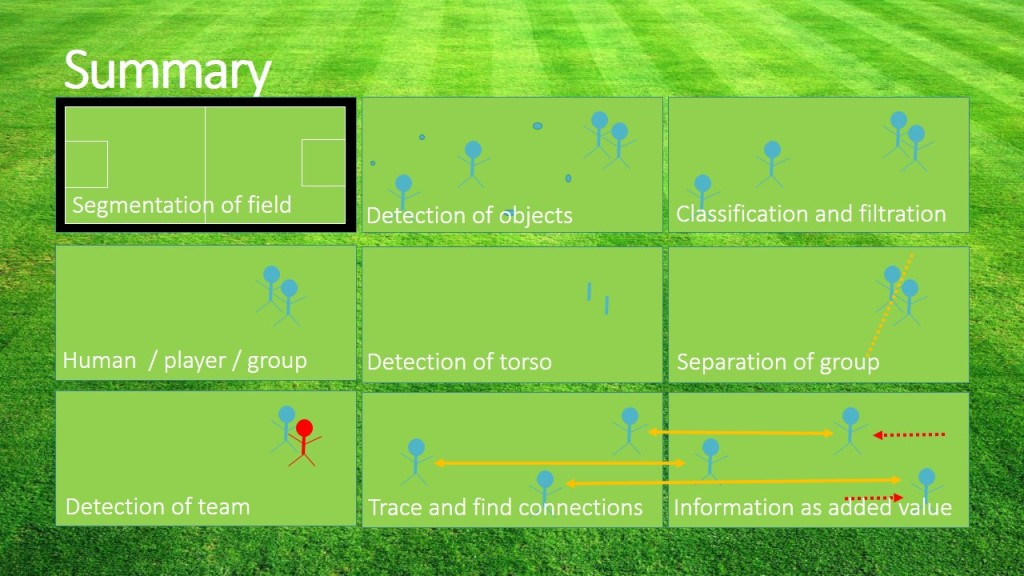Project repository:Â https://github.com/sekys/sk.seky.soccerball

Posted on

## Stereo reconstructionOndrej Galbavy

This example presents straightforward process to determine depth of points (sparse depth map) from stereo image pair using stereo reconstruction. Example is implemented in Python 2.

## Stereo calibration process

We need to obtain multiple stereo pairs with chessboard shown on both images.

1. For each stereo pair we need to do:
1. Find chessboard: cv2.findChessboardCorners
2. Find subpixel coordinates: cv2.cornerSubPix
3. If both chessboards are found, store keypoints
4. Optionally draw chessboard pattern on image
2. Compute calibraton: cv2.stereoCalibrate
1. We get:
1. Camera matrices and distortion coefficients
2. Rotation matrix
3. Translation vector
4. Essential and fundamental matrices
3. Store calibration data for used camera setup
```    for paths in calib_files:

left_img = cv2.imread(paths.left_path, cv2.CV_8UC1)
right_img = cv2.imread(paths.right_path, cv2.CV_8UC1)

image_size = left_img.shape

find_chessboard_flags = cv2.CALIB_CB_ADAPTIVE_THRESH | cv2.CALIB_CB_NORMALIZE_IMAGE | cv2.CALIB_CB_FAST_CHECK

left_found, left_corners = cv2.findChessboardCorners(left_img, pattern_size, flags = find_chessboard_flags)
right_found, right_corners = cv2.findChessboardCorners(right_img, pattern_size, flags = find_chessboard_flags)

if left_found:
cv2.cornerSubPix(left_img, left_corners, (11,11), (-1,-1), (cv2.TERM_CRITERIA_EPS + cv2.TERM_CRITERIA_MAX_ITER, 30, 0.1))
if right_found:
cv2.cornerSubPix(right_img, right_corners, (11,11), (-1,-1), (cv2.TERM_CRITERIA_EPS + cv2.TERM_CRITERIA_MAX_ITER, 30, 0.1))

if left_found and right_found:
img_left_points.append(left_corners)
img_right_points.append(right_corners)
obj_points.append(pattern_points)

cv2.imshow("left", left_img)
cv2.drawChessboardCorners(left_img, pattern_size, left_corners, left_found)
cv2.drawChessboardCorners(right_img, pattern_size, right_corners, right_found)

cv2.imshow("left chess", left_img)
cv2.imshow("right chess", right_img)

stereocalib_criteria = (cv2.TERM_CRITERIA_MAX_ITER + cv2.TERM_CRITERIA_EPS, 100, 1e-5)
stereocalib_flags = cv2.CALIB_FIX_ASPECT_RATIO | cv2.CALIB_ZERO_TANGENT_DIST | cv2.CALIB_SAME_FOCAL_LENGTH | cv2.CALIB_RATIONAL_MODEL | cv2.CALIB_FIX_K3 | cv2.CALIB_FIX_K4 | cv2.CALIB_FIX_K5
stereocalib_retval, cameraMatrix1, distCoeffs1, cameraMatrix2, distCoeffs2, R, T, E, F =        cv2.stereoCalibrate(obj_points,img_left_points,img_right_points,image_size,criteria = stereocalib_criteria, flags = stereocalib_flags)
```

## Stereo rectification process

We need to:

1. Compute rectification matrices: cv2.stereoRectify
2. Prepare undistortion maps for both cameras: cv2.initUndistortRectifyMap
3. Remap each image: cv2.remap
```rectify_scale = 0 # 0=full crop, 1=no crop
R1, R2, P1, P2, Q, roi1, roi2 = cv2.stereoRectify(data["cameraMatrix1"], data["distCoeffs1"], data["cameraMatrix2"], data["distCoeffs2"], (640, 480), data["R"], data["T"], alpha = rectify_scale)
left_maps = cv2.initUndistortRectifyMap(data["cameraMatrix1"], data["distCoeffs1"], R1, P1, (640, 480), cv2.CV_16SC2)
right_maps = cv2.initUndistortRectifyMap(data["cameraMatrix2"], data["distCoeffs2"], R2, P2, (640, 480), cv2.CV_16SC2)

for pair in pairs:
left_img_remap = cv2.remap(pair.left_img, left_maps, left_maps, cv2.INTER_LANCZOS4)
right_img_remap = cv2.remap(pair.right_img, right_maps, right_maps, cv2.INTER_LANCZOS4)
```

## Stereo pairing

Standard object matching: keypoint detection (Harris, SIFT, SURF,â€¦), descriptor extractor (SIFT, SURF) and matching (Flann, brute force,â€¦).Â  Matches are filtered for same line coordinates to remove mismatches.

```detector = cv2.FeatureDetector_create("HARRIS")
extractor = cv2.DescriptorExtractor_create("SIFT")
matcher = cv2.DescriptorMatcher_create("BruteForce")

for pair in pairs:
left_kp = detector.detect(pair.left_img_remap)
right_kp = detector.detect(pair.right_img_remap)
l_kp, l_d = extractor.compute(left_img_remap, left_kp)
r_kp, r_d = extractor.compute(right_img_remap, right_kp)
matches = matcher.match(l_d, r_d)
sel_matches = [m for m in matches if abs(l_kp[m.queryIdx].pt - r_kp[m.trainIdx].pt) &lt; 3]
```

## Triangulation

How do we get depth of point? Dispartity is difference of x coordinate of the same keypoint in both images. Closer points have greater dispartity and far points have almost zero dispartity. Depth can be defined as: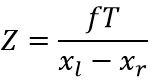Where:

• f â€“ focal length
• T â€“ baseline â€“ distance of cameras
• x1, x2 â€“ x coordinated of same keypoint
• Z â€“ depth of point
```for m in sel_matches:
left_pt = l_kp[m.queryIdx].pt
right_pt = r_kp[m.trainIdx].pt
dispartity = abs(left_pt - right_pt)
z = triangulation_constant / dispartity
```

Posted on

## Motion Analysis & Object TrackingPavol Zbell

## Introduction

In our work we focus on basics of motion analysis and object tracking. We compare MeanShift (non-parametric, finds an object on a back projection image) versus CamShift (continuously adaptive mean shift, finds an object center, size, and orientation) algorithms and effectively utilize them to perform simple object tracking. In case these algorithms fail to track the desired object or the object travels out of window scope, we try to find another object to track. To achieve this, we use a background subtractor based on a Gaussian Mixture Background / Foreground Segmentation AlgorithmÂ  to identify the next possible object to track. There areÂ  two suitable implementations of this algorithm in OpenCV â€“ BackgroundSubtractorMOG and BackgroundSubtractorMOG2. We also compare performance of both these implementations.

Used functions:Â calcBackProject, calcHist, CamShift, cvtColor, inRange, meanShift, moments, normalize

## Solution

1. Initialize tracking window:
• Set tracking window near frame center
2. Track object utilizing MeanShift / CamShift
• Calculate HSV histogram of region of interest (ROI) and track
```int dims = 1;
int channels[] = {0};
int hist_size[] = {180};
float hranges[] = {0, 180};
const float *ranges[] = {hranges};
roi = frame(track_window);
cvtColor(roi, roi_hsv, cv::COLOR_BGR2HSV);
// clamp > H: 0 - 180, S: 60 - 255, V: 32 - 255
inRange(roi_hsv, Scalar(0.0, 60.0, 32.0), Scalar(180.0, 255.0, 255.0), mask);
calcHist (&roi_hsv, 1, channels, mask, roi_hist, dims, hist_size, ranges);
normalize(roi_hist, roi_hist, 0, 255, NORM_MINMAX);
...
Mat hsv, dst;
cvtColor(frame, hsv, cv::COLOR_BGR2HSV);
calcBackProject(&hsv, 1, channels, roi_hist, dst, ranges, 1);
clamp_rect(track_window, bounds);
print_rect("track-window", track_window);
Mat result = frame.clone();
if (use_camshift) {
RotatedRect rect = CamShift(dst, track_window, term_criteria);
draw_rotated_rect(result, rect, Scalar(0, 0, 255));
}
else {
meanShift(dst, track_window, term_criteria);
draw_rect(result, track_window, Scalar(0, 0, 255));
}
```
3. Lost tracked object?
• In other words, is the centeroid of MOG mask out of tracking window?
```bool contains;
if (use_camshift) {
contains = rect.boundingRect().contains(center);
}
else {
contains = center.inside(track_window);
}
```
4. When lost, reinitialize tracking window:
• Set tracking window to centeroid of MOG mask
• Go back to 2. and repeat
```mog->operator()(frame, mask);
track_window = RotatedRect(Point2f(center.x, center.y), Size2f(100, 50), 0).boundingRect();
```

## Samples

As seen on Fig.Â 1, MeanShift (left) operates with fixed size tracking windows which can not be rotated. On the contrary, CamShift (right) utilizes the full potential of dynamic size rotated rectangles. Working with CamShift yielded significantly better tracking results in general. On the other hand we recommend to use MeanShift when the object is in constant distance from the camera and moves without rotation (or is represented by a circle), in such case MeanShift performs faster than CamShift and produces sufficient results without any rotation or size change noise.

Comparison of BackgroundSubtractorMOG and BackgroundSubtractorMOG2 is depicted on Fig. 2.Â MOG approach is simpler than MOG2 as it considers only binary masks whereas MOG2 operatesÂ on a full gray scale masks. Experiments shown that in our specific case MOG performed better as itÂ yielded less information noise than MOG2. MOG2 will probably produce better results than MOGÂ when utilized more effectively than in out initial approach (simple centeroid from mask extraction).

## Summary

In this project explored the possibilities of simple object tracking via OpenCV APIs utilizingÂ various algorithms such as MeanShift and CamShift, Background Extractor MOG and MOG2,Â which we also compared. Our solution performs relatively well, but we can certainly improve it byÂ fine tuning histogram calculation, MOG, and other parameters. Other improvements can be done inÂ MOG usage, as now the objects are only recognized by finding MOG mask centeroids. This alsoÂ calls to better tracking window initialization process.

Posted on

## Signature recognitionMatej Stetiar

Main purpose of this project was to recognise signatures. For this purpose we used descriptor from the bottom of the signature. Then we used Mahalanobis distance to identify signatures.

## Image preprocessing

We have worked with 2 sets of signatures and each of them had about 200 pictures of signatures. Examples of those signatures are below.

The signatures had different quality. So we decided to find skeleton of them.

```Mat skel(tmp_image.size(), CV_8UC1, Scalar(0));
Mat tmp(tmp_image.size(), CV_8UC1);

Mat structElem = getStructuringElement(MORPH_CROSS, Size(3, 3));

do
{
morphologyEx(tmp_image, tmp, MORPH_OPEN, structElem);
bitwise_not(tmp, tmp);
bitwise_and(tmp_image, tmp, tmp);
bitwise_or(skel, tmp, skel);
erode(tmp_image, tmp_image, structElem);

double max;
minMaxLoc(tmp_image, 0, &max);
done = (max == 0);
} while (!done);
```

Then we decided to find contours and filter them according to their size to remove the noise.

```vector<vector<Point>> contours;
vector<Vec4i> hierarchy;

findContours(image, contours, hierarchy, CV_RETR_TREE, CV_CHAIN_APPROX_SIMPLE);

Mat drawing = Mat::zeros(image.size(), CV_8UC1);
for (int i = 0; i< contours.size(); i++){
if (contours[i].size() < 10.0) continue;
Scalar color = Scalar(255);
drawContours(drawing, contours, i, color, CV_FILLED, 8, hierarchy);
}
```

The result of image preprocessed like this can be seen below. This picture has very thick lines so we decided to add contour image and skeleton image together.

For purpose of adding 2 images together we used logical function AND.

```bitwise_and(newImage, skeleton, contours);
```

The result of this process was signature with thin line with no noise.

## Creating descriptors

To create descriptors we used bottom line of the signature. To lower the factor of length of signature we always divided signature to 25 similar pieces. Space between these pieces was calculated dynamically. The descriptor was gathered as maximum of white point position in each of 25 division points.

To reduce the factor that signature is written in some angle we transformed the points to lower positions. To do so we gathered points in 10Â° range from lowest point. Then we calculated average of these points. Then we used linear regression to add coefficient to all points. Linear regression was made using maximal point and average of the other points.

Descriptor we created had counted with different lengths of signatures and different angles of signatures. So the last step was to normalise the height. To do so we subtracted minimum point of the signature from all points and then we divided all points with maximum from descriptor.

## Learning phase

We created 2 sets of descriptors each with 180 examples. From these descriptors we created 2 objects of class Signature.

```class Signature
{
std::string name; //signature name
cv::Mat centorid; //centorid created from learning set
cv::Mat covarMat; //covariance matrix created form lenrning set
};
```

To recognition of the signatures we wanted to use Mahalanobis distance method. To do so we needed centroid for our data set and inverse covariance matrix. We calculated those using functions:

```cv::calcCovarMatrix(samples, this->covarMat, this->centorid, CV_COVAR_NORMAL | CV_COVAR_ROWS);
cv::invert(this->covarMat, this->covarMat, cv::DECOMP_SVD);
```

In code above the variable samples is representing the matrix of all samples.

## Testing

After we created inverse covariance matrix and centroid we could start testing. Testing of the signature was creating its descriptor using same steps as when creating descriptors in testing set. Then we could call function to calculate Mahalanobis distance.

```Mahalanobis(testSample, this->mean, this->covarMat);
```

Using this algorithm we were able to identify some of the signatures. But the algorithm is very sensitive to changes in image quality and number of items in training set.

Posted on

## Tracking moving objectThis example shows how to separate and track moving object using OpenCV. First, the background of the video is being calculated and moving objects detected, then it is filtered and tracked.

Used: cv::BackgroundSubtractorMOG2; cv::getStructuringElement; cv::morphologyEx; cv::BackgroundSubtractorMOG2.operator();

## The process

1. Initialize the background extraction object
```BackgroundSubtractorMOG2 bg( 500, 64, false);
```
2. Process video frame by background extraction object by it’s method operator and receive mask of moving object
```bg.operator()( origi, mask);
```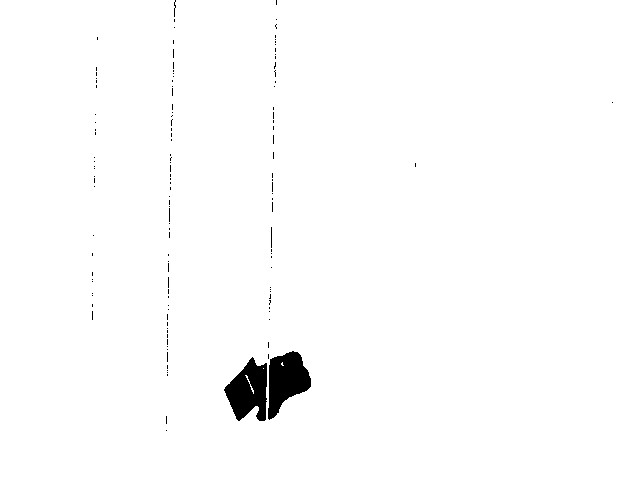3. Process mask by morphologyEx’s open to remove noise in mask
```morphologyEx( mask, mask, MORPH_OPEN, element1 );
```
4. Process mask by morphologyEx’s close to close gaps in mask
```morphologyEx( mask, mask, MORPH_CLOSE, element2 );
```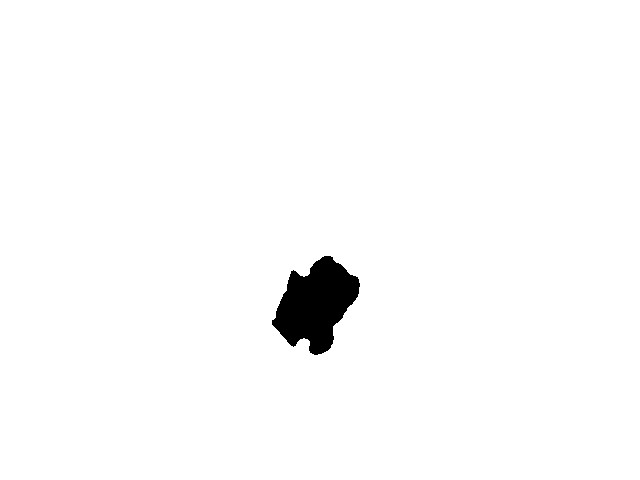5. Apply mask on video frame
```origi.copyTo( proci0, mask);
```
6. Find good features to track and apply KLTracker
```goodFeaturesToTrack( proci0, points, MAX_COUNT, 0.1, 10, Mat(), 3, 0, 0.04);
calcOpticalFlowPyrLK( proci1, proci0, points, points, status, err, winSize, 3, termcrit, 0, 0.00001);
```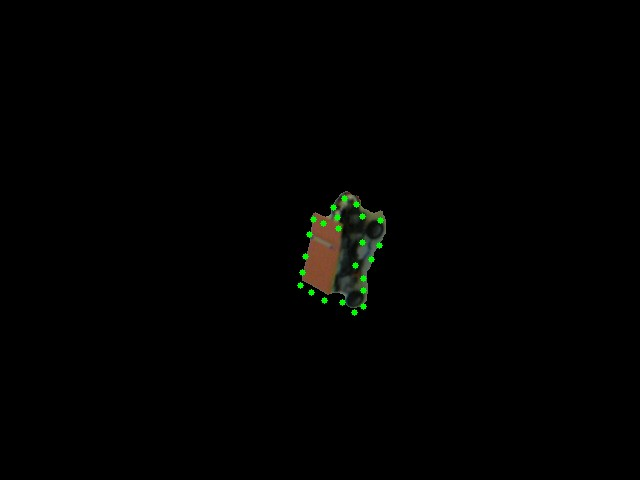7. Combine with initial frame
```size_t i, k;
for (i = k = 0; i &lt; points.size(); i++)
{
if (!status[i]) continue;
points[k++] = points[i];
circle(finI, points[i], 3, Scalar(0, 255, 0), -1, 8);
circle(procI2, points[i], 3, Scalar(0, 255, 0), -1, 8);
}
points.resize(k);
```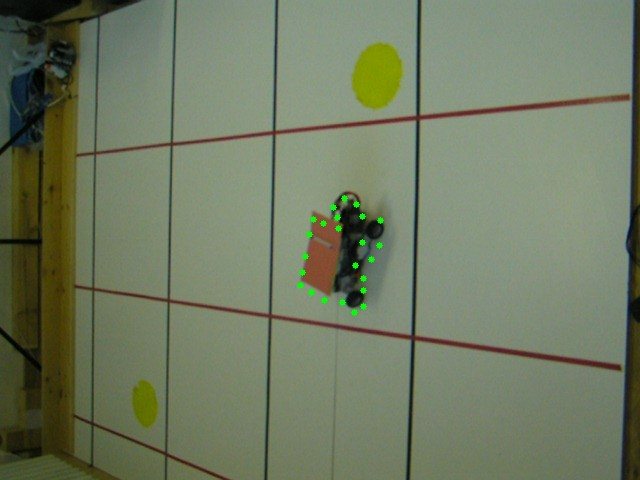8. Find contours of the mask, find it’s bounding rectangle and draw it onto output frame
```findContours(procI3, contours, CV_RETR_LIST, CV_CHAIN_APPROX_SIMPLE);
if (contours.size() &gt; 0)
for (int i = 0; i &lt; (int)contours.size(); i++)
rectangle(finI, boundingRect(contours[i]), Scalar(0, 255, 0));
```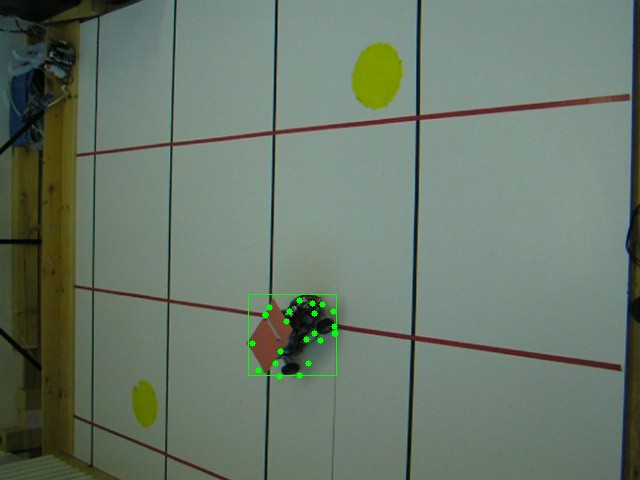Bounding rectangle hints position of moving object on the scene and could be used to approximate it’s coordinates

Posted on

## Object removing in image/videoMarek Grznar

## Introduction

In our project we focus on simple object recognition, then tracking this recognized object and finally we try to delete this object from video. By object recognition we used local features-based methods. We compare SIFT and SURF methods for detection and description. By RANSAC algorithm we compute the homography. In case these algorithms successfully find the object we create a mask where recognized object was white area and the rest was black. By object tracking we compared the two approaches. The first approach is based on calculating optical flow using the iterative Lucas-Kanade method with pyramids. The second approach is based on camshift tracking algorithm. For deleting the object from video we focus to using algorithm based on restoring the selected region in an image using the region neighborhood.

Used functions: floodFill, findHomography, match, fillPoly, goodFeaturesToTrack, calcOpticalFlowPyrLK, inpaint, mixChannels, calcHist, CamShift

## Solution

1. Opening video file, retrieve the next frame (picture), converting from color image to grayscale
```cap.open("Video1.mp4");
cap >> frame;
frame.copyTo(image);
cvtColor(image, gray, COLOR_BGR2GRAY);
```
2. Find object in frame (picture)
1. Keypoints detection and description (SIFT/SURF)
```// SiftFeatureDetector detector( minHessian );
SurfFeatureDetector detector( minHessian );

std::vector<KeyPoint> keypoints_object, keypoints_scene;

detector.detect(img_object, keypoints_object);
detector.detect(img_scene, keypoints_scene);

// SiftDescriptorExtractor extractor;
SurfDescriptorExtractor extractor;

Mat descriptors_object, descriptors_scene;

extractor.compute(img_object, keypoints_object, descriptors_object);
extractor.compute(img_scene, keypoints_scene, descriptors_scene);
```
2. Matching keypoints
```FlannBasedMatcher matcher;
std::vector< DMatch > matches;
matcher.match( descriptors_object, descriptors_scene, matches );
```
3. Homography calculating
```Mat H = findHomography( obj, scene, CV_RANSAC );
```
```cv::Mat mask(img_scene.size().height,img_scene.size().width,CV_8UC1);
cv::fillPoly(mask,&pts, &n, 1, Scalar::all(255));
```

### First tracking approach

1. Find significant points in current frame (using mask with recognized object)
2. Find significant points from the previous frame to the next
3. Deleting object from image
1. Calculate mask of current object position
2. Modify mask of current object position
3. Restore the selected region in an image using the region neighborhood.

### Second tracking approach

1. Calculate histogram of ROI
2. Calculate the back projection of histogram
3. Track object using camshift

## Object recognition

### Input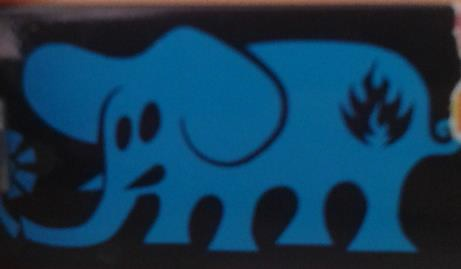## Tracking object

### Input (tracked object)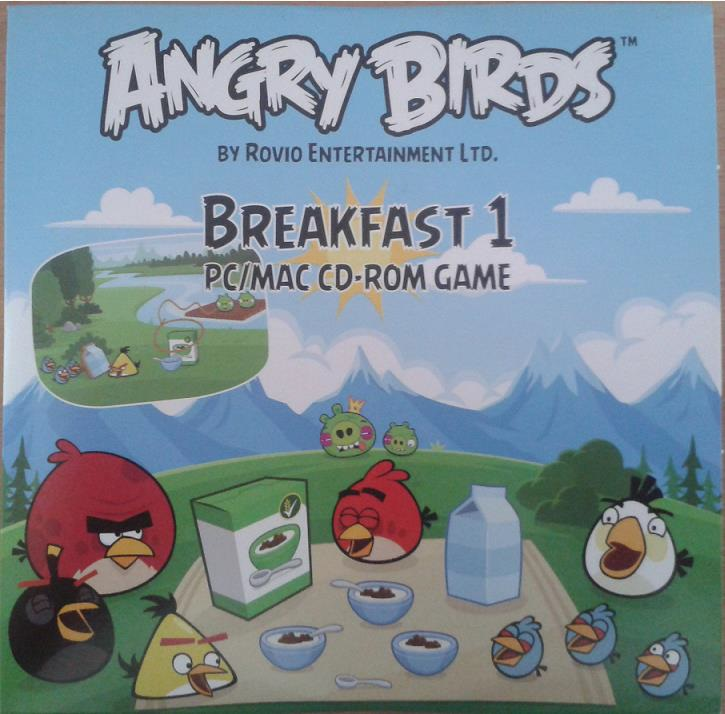## Modifying mask for deleting object

### Input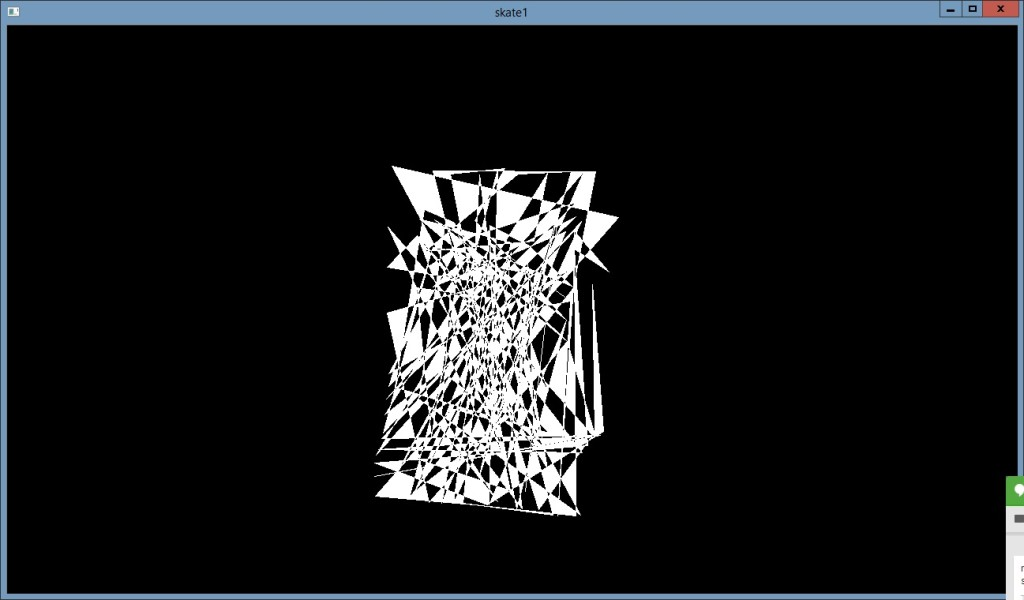### Output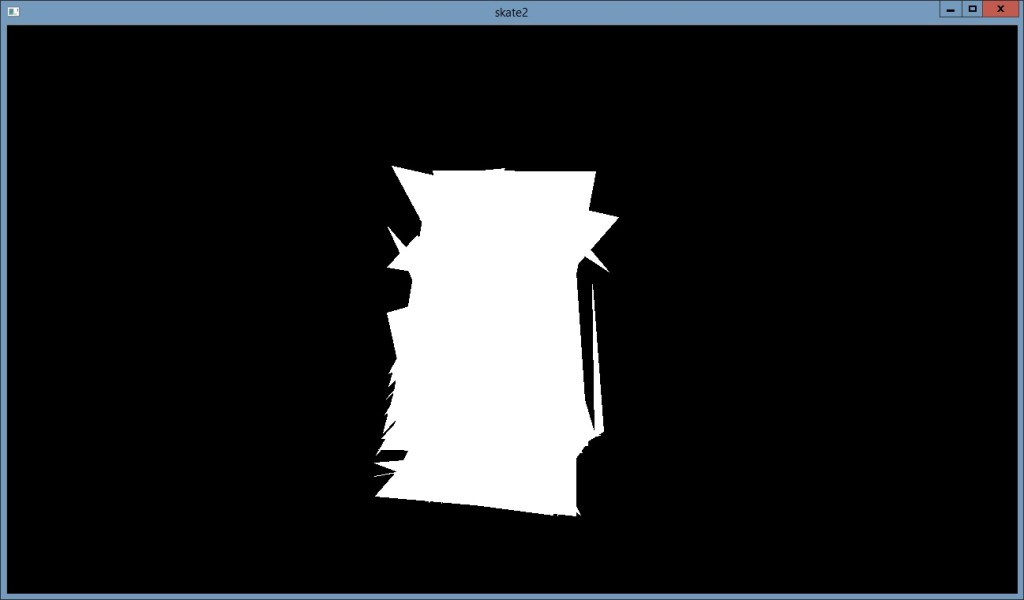## Deleting object

### Input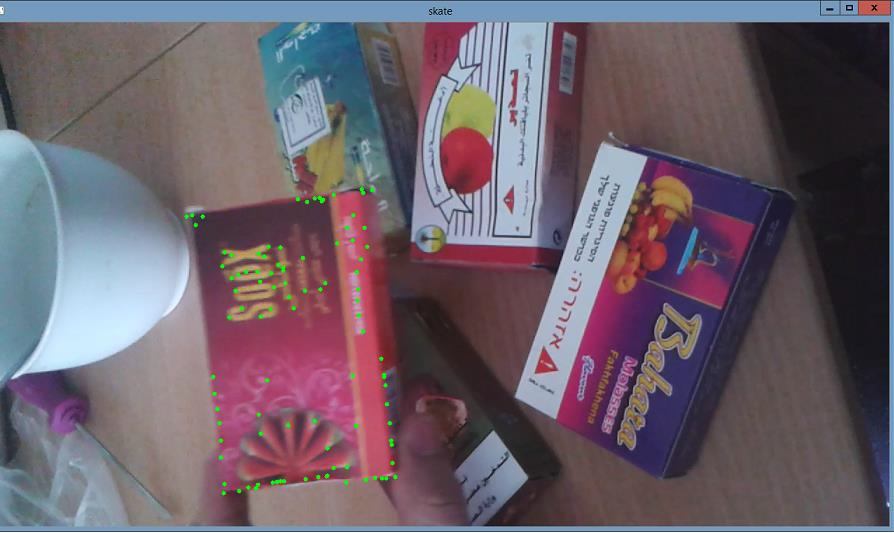### Output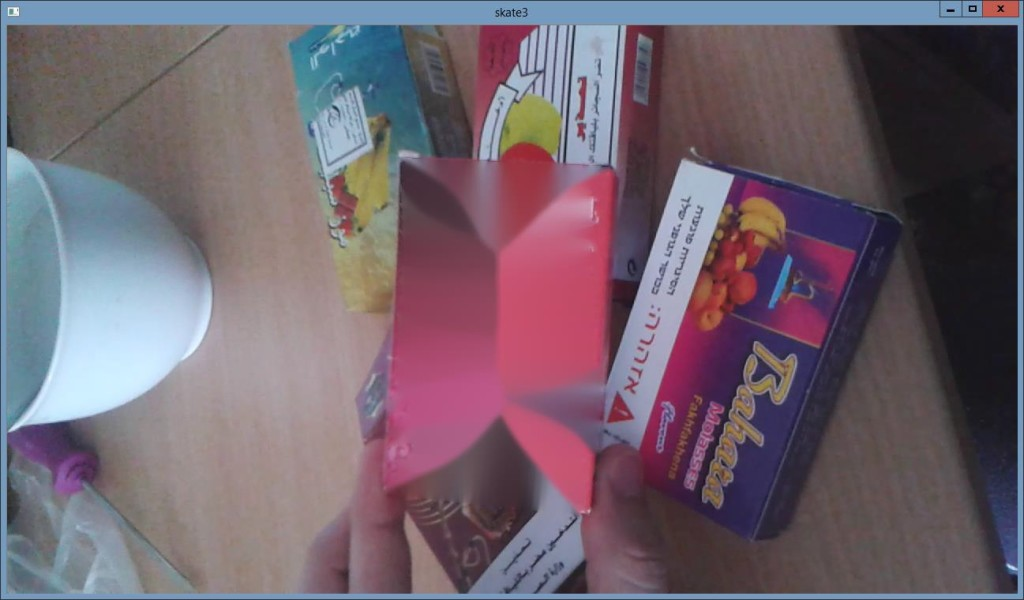Posted on

## Local Descriptors in OpenCvTomas Martinkovic

The project shows detection of chocolate cover from input image or frame of video. For each video orÂ image may be chosen various combinations of detector with descriptor. For matching object ofÂ chocolate cover with input frame or image automatically is used FlannBasedMatcher orÂ BruteForceMatcher. It depends on the chosen SurfDescriptorExtractor or FREAK algorithm.

Functions used: SurfFeatureDetector, FastFeatureDetector, SiftFeatureDetector, StarFeatureDetector,Â SurfDescriptorExtractor, FREAK, FlannBasedMatcher, BruteForceMatcher, findHomography

## Process

1. Preprocessing â€“ Conversion to grayscale
```cvtColor(frame, img_scene, CV_BGR2GRAY);
```
2. Detect the keypoints
```detector_Surf = getSurfFeatureDetector();
detector_Surf.detect( img_object, keypoints_object );
detector_Surf.detect( img_scene, keypoints_scene );
```
3. Compute local descriptors
```extractor_freak.compute( img_object, keypoints_object, descriptors_object );
extractor_freak.compute( img_scene, keypoints_scene, descriptors_scene );
```
4. Matching local descriptors
```BruteForceMatcher<Hamming> matcher;
matcher.match( descriptors_object, descriptors_scene, matches );
```
5. Draw good matches to frame
```drawMatches( img_object, keypoints_object, img_scene, keypoints_scene, good_matches, img_matches, Scalar::all(-1), Scalar::all(-1), vector<char>(), DrawMatchesFlags::NOT_DRAW_SINGLE_POINTS );
```
6. Finding homography and drawing frame of video
```Mat H = findHomography( obj, scene, CV_RANSAC );
perspectiveTransform( obj_corners, scene_corners, H);
imshow( "Object detection on video", img_matches );
```